International Journal of High Energy Physics
Volume 2, Issue 4, August 2015, Pages: 47-49

Higgs Masses in a Renormalization-GroupImproved Non-Minimal Model of Supersymmetry

Partha Pratim Pal1, Biswanath Ghosh2

1Bolpur sikshaniketan Ashram Vidyalaya, Bolpur, Birbhum, West Bengal, India

2Paschimsundalpur High School, Netaipur, Birbhum, West Bengal, India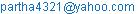(P. P. Pal)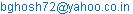(B. Ghosh)

Partha Pratim Pal, Biswanath Ghosh. Higgs Masses in a Renormalization-GroupImproved Non-Minimal Model of Supersymmetry. International Journal of High Energy Physics. Vol. 2, No. 4, 2015, pp. 47-49. doi: 10.11648/j.ijhep.20150204.11

Abstract: We give a systematic study of Higgs masses in a non-minimal model of supersymmetry with renormalization group improvement for extended values of the parameters like tanβ , MC (the mass of charged Higgs) and Q (the squark mass scale), in the context of LHC experiments. Several new and interesting results are obtained.

Keywords: Higgs Mass, Non-Minimal Model of Supersymmetry, Renormalization Group, LHC Experiment

1. Introduction

Supersymmetry is a very important tool for studying physics beyond standard model . It is being vigorously pursued for detecting supersymmetric particles in nature. The supersymmetric particles include the supersymmetric partners of ordinary particles and supersymmetric Higgs bosons. The minimal supersymmetric standard model (MSSM) , for example, contain two CP-even neutral Higgs bosons, a CP-odd neutral Higgs boson and two charged Higgs bosons. The relaxation of any of the assumptions of MSSM leads to non-minimal model (NMSSM). Ellis et al  gave non-minimal model by inclusion of a single Higgs field whose vacuum expectation value determined the mixing of the two Higgs doublet of the minimal supersymmetric standard model. These authors studied the spectrum and couplings of Higgs bosons in this extended model and compared them with those in the minimal model. In their extensive work , Ellis et al analyzed the possible production mechanisms and phenomenological signatures of the different Higgs bosons at the TeV-scale.

On the other hand, Pandita  has calculated the dominant one-loop radiative corrections arising from quark-squark loops to the mass squared matrix of the CP-even Higgs bosons in a non-minimal supersymmetric standard model containing two Higgs doublets and a Higgs singlet chiral superfield using one-loop effective potential approximation. He evaluated upper and lower bounds on the radiatively corrected masses of all the scalar Higgs bosons as a function of parameters of the model. He found that the one-loop radiative corrections were substantial only for the lightest Higgs boson of the model. He also calculated an absolute upper bound on the mass of the radiatively corrected lightest Higgs boson and compared it with the corresponding bound in the MSSM. Other interesting works on Higgs in NMSSM exist in the literature .

The present study is different from previous study  in some aspects. For example, we do not consider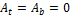but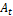and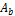are given by non-zero values according to the Renormalization group improvement . We consider the value of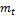given by the experimental value of 174 GeV. We consider dependence of the Higgs masses on the values ofwhich varies from 0-50, unlike the previous work . Moreover the charged Higgs mass is varied from 0-1000 GeV and supersymmetry breaking scale is considered to be very large (1 TeV).

The paper is organized as follows: Section 2 gives the expressions of Higgs masses in non-minimal supersymmetric standard model. In section 3 we give the renormalization group improvement  that is to be incorporated into the expression for the Higgs mass. The results and discussions are given in section 4. The conclusions are given in section 5.

2. Higgs masses in Non-Minimal Supersymmetric Standard Model

Following ref. , the radiatively corrected mass-squared matrix for the CP-even mass eigenstates are given by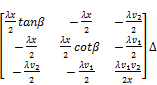(1)

Where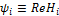for i=1,2 and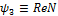. The eigenvalues of this matrix are the Higgs square masses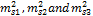.The eigenvalues ofandmass squared matrices are given by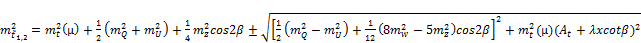(2)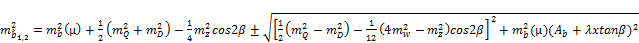(3)

Where the approximation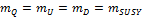and the relations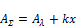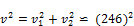(4)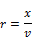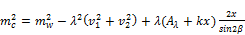(5)

and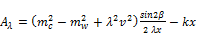(6)

are used.

3. RG-improvement of Yukawa Couplings

The renormalization group (RG) equations for the top-quark and bottom-quark Yukawa couplings are given by :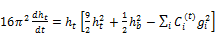(7)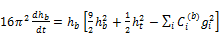(8)

where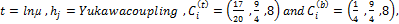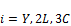.

We now introduce, in brief, the method of solution of RG-equations  which has been used in our analysis. For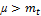, the integration of equations (7,8) gives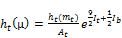(9)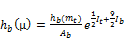(10)

where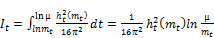(11)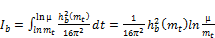(12)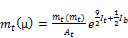(13)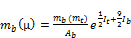(14)

and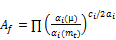(15)

The functions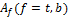are obtained byintegrating out the gauge coupling contributions in equations (7,8) and keeping terms up to one loop out of the two loop approximation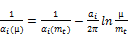(16)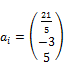(17)

Here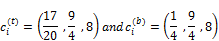For a given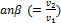, the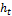and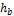Yukawa couplings are computed at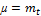using the following relations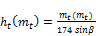(18)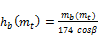(19)

The equations (9-19) are additional improvisation to the earlier work of ref. giving the RG-modified Higgs masses.

4. Results and Discussions

The numerical values of parameters chosen by us in this model are as follows: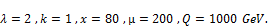In our systematic study, the variation of Higgs boson masses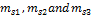withare studied for a wide range of variation of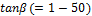for a particular value of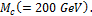We find thatcan have a minimum value of about 200 GeV and a maximum value of about 390 GeV. The corresponding values of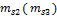can be about 260 (550) GeV and 390 (900) GeV. Interestingly, we also find that the minimum values of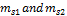corresponds to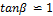. For this value ofis about 900 GeV. The highest values ofcorrespond to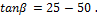For large values of, the value of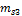is about 550 GeV.

Next the variation ofwith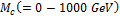for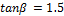and Q =1 TeV are studied for the parameters chosen in ref.  (viz.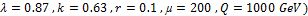after RG –improvement to the model. The minimum value ofis about 25 GeV (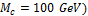and maximum value ofis 180 GeV forand Q =1 TeV. For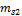the minimum value is about 250 GeV (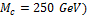and forthe minimum value is 780 GeV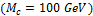for sameand Q.

These results suggest that RG-improvements on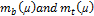give rather large values ofcompared to the predictions on the upper limit (~150-160 GeV) in NMSSM [8, 9, 10]. Besides this, the upper bound on the mass of the lightest Higgs boson studied in this paper is larger than that in SM  and MSSM. However, the studies considered in this paper will hopefully be verified in the future LHC experiments.

5. Conclusions

This simple-minded study includes the effect of RG-improvement to the Higgs masses in NMSSM . Unlike the previous studies [3,4], one considers here the experimental value of the top quark mass and an appropriate value of the squark mass scale. Moreover the variation ofwithare also studied here. This study also includes the parameters given in ref.. These analyses give some new results for large.

Thus the RG-improvements in the Yukawa couplings of the b-and t-quarks introduce certain interesting features in the analyses of the Higgs masses in non-minimal supersymmetric standard model. We find that the Higgs masses are now much heavier than the previous results and are most likely to be discovered in the mass range covered by the LHC.

References

1. H.P.Nilles, Phys.Rep. 110(1984)1; H.E.Haber and G.L.Kane, Phys. Rep. 117 (1985) 75; A Lahyanas and D.V. Nanopoulos, Phys.Rep. 145 (1987)1; J.L.Lopez, D.V. Nanopoulos and A. Zichichi, Prog. in Part. and Nucl.Phys.33 (1994) 303.
2. Howard E. Haber, Nucl. Phys. B(proc.suppl.) 62 A-C (1998) 469 and references there in.
3. J. Ellis, J.F. Gunion, H.E. Haber, L.Roszkowski and F.Zwirner, Phys.Rev. D39(1989)844.
4. P. N. Pandita, Z. Phys. C 59 (1993) 575.
5. U. Ellwanger, Natural Range of Higgs Masses in Supersymmetry, Preprint CERN- TH 6144/91, HD-THEP-91-21 Sept. (1991).
6. M. K. Parida and A. Usmani, Phys. Rev. D 54 (1996) 3663.
7. H. E. Haber and R. Hempling, Phys. Rev. Lett. 66 (1991) 1815; H. E. Haber, R. Hempling and A.H. Hoang, Z. Phys. C 75 (1997) 539 .
8. Partha Pratim Pal, InternationalJournal of High Energy Physics, Vol. 2, No. 3, 2015, pp. 34-37.
9. D. J. Miller, R. Nevzorov and P. M. Zerwas, Nucl. Phys. B 681, 3 (2004).
10. J. Ellis, J. F. Gunion, H. Haber, L. Roszkowski, F. Zwirner, Phys. Rev. D 39 (1989) 844.
11. P.P.Pal, American Journal of Physics and applications, 2015 ; 3(2) :63-66. DOI:10. 11648/j. ajpa. 20150302. 18.
12. P.P.Pal, American Journal of Physics and applications, 2015 ; 3(2) :67-59. DOI:10. 11648/j. ajpa. 20150302. 16.

 Contents 1. 2. 3. 4. 5.
Article ToolsAbstractPDF(172K)